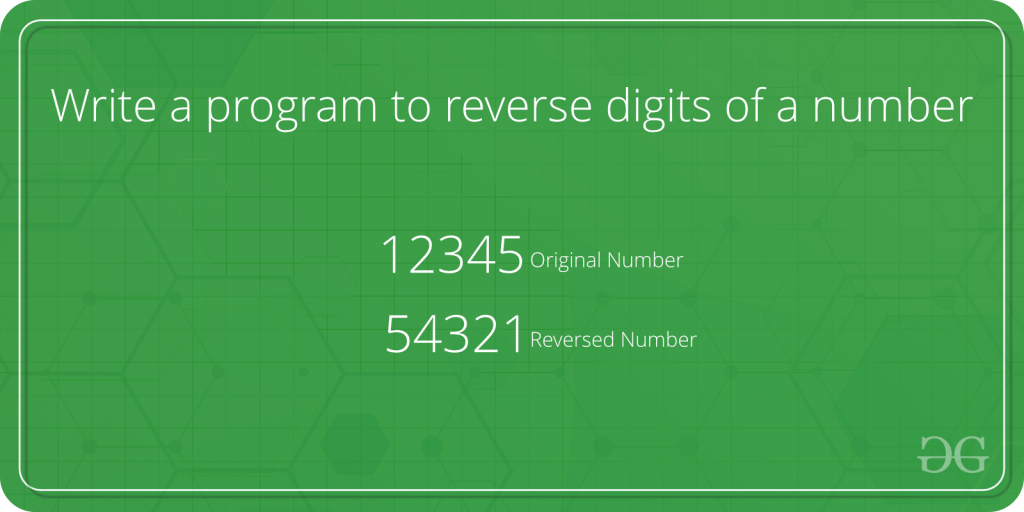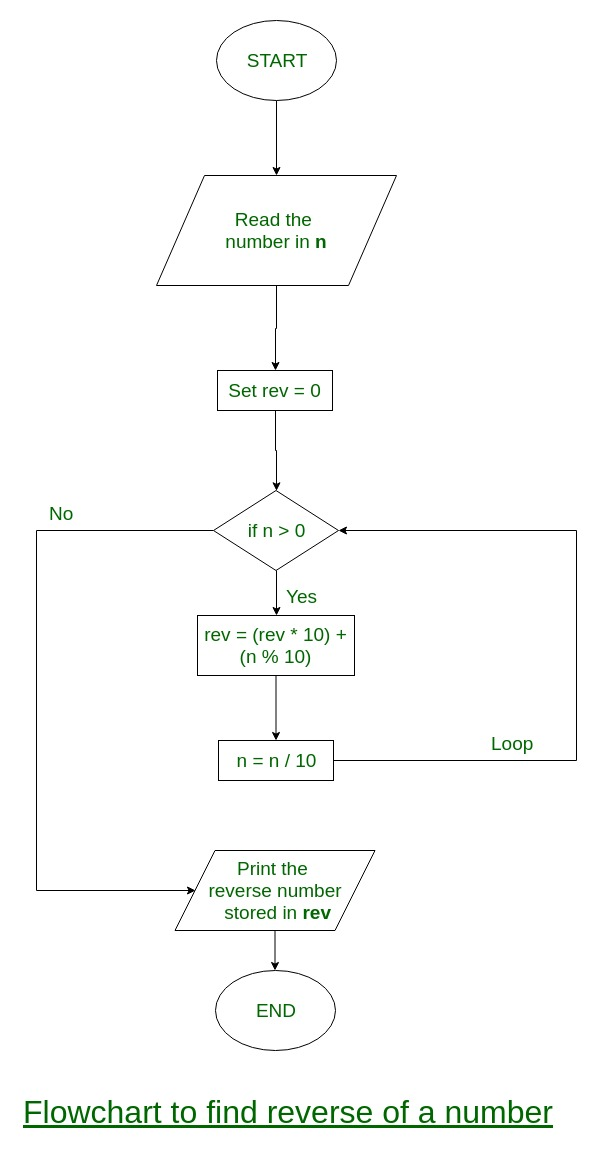Skip to content
Related Articles
Write a program to reverse digits of a number
• Difficulty Level : Easy
• Last Updated : 02 Jun, 2021

Write a program to reverse the digits of an integer.Examples :

```Input : num = 12345
Output: 54321

Input : num = 876
Output: 678```

Flowchart:ITERATIVE WAY

Algorithm:

```Input:  num
(1) Initialize rev_num = 0
(2) Loop while num > 0
(a) Multiply rev_num by 10 and add remainder of num
divide by 10 to rev_num
rev_num = rev_num*10 + num%10;
(b) Divide num by 10
(3) Return rev_num```

Example:

num = 4562
rev_num = 0
rev_num = rev_num *10 + num%10 = 2
num = num/10 = 456
rev_num = rev_num *10 + num%10 = 20 + 6 = 26
num = num/10 = 45
rev_num = rev_num *10 + num%10 = 260 + 5 = 265
num = num/10 = 4
rev_num = rev_num *10 + num%10 = 2650 + 4 = 2654
num = num/10 = 0

Program:

## C++

 `#include ` `using` `namespace` `std;``/* Iterative function to reverse digits of num*/``int` `reversDigits(``int` `num)``{``    ``int` `rev_num = 0;``    ``while` `(num > 0) {``        ``rev_num = rev_num * 10 + num % 10;``        ``num = num / 10;``    ``}``    ``return` `rev_num;``}` `/*Driver program to test reversDigits*/``int` `main()``{``    ``int` `num = 4562;``    ``cout << ``"Reverse of no. is "` `<< reversDigits(num);``    ``getchar``();``    ``return` `0;``}` `// This code is contributed``// by Akanksha Rai(Abby_akku)`

## C

 `#include ` `/* Iterative function to reverse digits of num*/``int` `reversDigits(``int` `num)``{``    ``int` `rev_num = 0;``    ``while` `(num > 0) {``        ``rev_num = rev_num * 10 + num % 10;``        ``num = num / 10;``    ``}``    ``return` `rev_num;``}` `/*Driver program to test reversDigits*/``int` `main()``{``    ``int` `num = 4562;``    ``printf``(``"Reverse of no. is %d"``, reversDigits(num));` `    ``getchar``();``    ``return` `0;``}`

## Java

 `// Java program to reverse a number` `class` `GFG {``    ``/* Iterative function to reverse``    ``digits of num*/``    ``static` `int` `reversDigits(``int` `num)``    ``{``        ``int` `rev_num = ``0``;``        ``while` `(num > ``0``) {``            ``rev_num = rev_num * ``10` `+ num % ``10``;``            ``num = num / ``10``;``        ``}``        ``return` `rev_num;``    ``}` `    ``// Driver code``    ``public` `static` `void` `main(String[] args)``    ``{``        ``int` `num = ``4562``;``        ``System.out.println(``"Reverse of no. is "``                           ``+ reversDigits(num));``    ``}``}` `// This code is contributed by Anant Agarwal.`

## Python

 `# Python program to reverse a number` `n ``=` `4562``rev ``=` `0` `while``(n > ``0``):``    ``a ``=` `n ``%` `10``    ``rev ``=` `rev ``*` `10` `+` `a``    ``n ``=` `n ``/``/` `10` `print``(rev)` `# This code is contributed by Shariq Raza`

## C#

 `// C# program to reverse a number``using` `System;` `class` `GFG {``    ``// Iterative function to``    ``// reverse digits of num``    ``static` `int` `reversDigits(``int` `num)``    ``{``        ``int` `rev_num = 0;``        ``while` `(num > 0) {``            ``rev_num = rev_num * 10 + num % 10;``            ``num = num / 10;``        ``}``        ``return` `rev_num;``    ``}` `    ``// Driver code``    ``public` `static` `void` `Main()``    ``{``        ``int` `num = 4562;``        ``Console.Write(``"Reverse of no. is "``                      ``+ reversDigits(num));``    ``}``}` `// This code is contributed by Sam007`

## PHP

 ` 1)``    ``{``        ``\$rev_num` `= ``\$rev_num` `* 10 +``                        ``\$num` `% 10;``        ``\$num` `= (int)``\$num` `/ 10;``    ``}``    ``return` `\$rev_num``;``}` `// Driver Code``\$num` `= 4562;``echo` `"Reverse of no. is "``,``       ``reversDigits(``\$num``);` `// This code is contributed by aj_36``?>`

## Javascript

 ``
Output
`Reverse of no. is 2654`

Time Complexity: O(log(n)), where n is the input number.

RECURSIVE WAY

## C++

 `// C++ program to reverse digits of a number``#include ``using` `namespace` `std;``/* Recursive function to reverse digits of num*/``int` `reversDigits(``int` `num)``{``    ``static` `int` `rev_num = 0;``    ``static` `int` `base_pos = 1;``    ``if` `(num > 0) {``        ``reversDigits(num / 10);``        ``rev_num += (num % 10) * base_pos;``        ``base_pos *= 10;``    ``}``    ``return` `rev_num;``}` `// Driver Code``int` `main()``{``    ``int` `num = 4562;``    ``cout << ``"Reverse of no. is "` `<< reversDigits(num);` `    ``return` `0;``}` `// This code is contributed``// by Akanksha Rai(Abby_akku)`

## C

 `// C program to reverse digits of a number``#include ;` `/* Recursive function to reverse digits of num*/``int` `reversDigits(``int` `num)``{``    ``static` `int` `rev_num = 0;``    ``static` `int` `base_pos = 1;``    ``if` `(num > 0) {``        ``reversDigits(num / 10);``        ``rev_num += (num % 10) * base_pos;``        ``base_pos *= 10;``    ``}``    ``return` `rev_num;``}` `/*Driver program to test reversDigits*/``int` `main()``{``    ``int` `num = 4562;``    ``printf``(``"Reverse of no. is %d"``, reversDigits(num));` `    ``getchar``();``    ``return` `0;``}`

## Java

 `// Java program to reverse digits of a number` `// Recursive function to``// reverse digits of num``class` `GFG {``    ``static` `int` `rev_num = ``0``;``    ``static` `int` `base_pos = ``1``;``    ``static` `int` `reversDigits(``int` `num)``    ``{``        ``if` `(num > ``0``) {``            ``reversDigits(num / ``10``);``            ``rev_num += (num % ``10``) * base_pos;``            ``base_pos *= ``10``;``        ``}``        ``return` `rev_num;``    ``}` `    ``// Driver Code``    ``public` `static` `void` `main(String[] args)``    ``{``        ``int` `num = ``4562``;``        ``System.out.println(reversDigits(num));``    ``}``}` `// This code is contributed by mits`

## Python3

 `# Python 3 program to reverse digits``# of a number``rev_num ``=` `0``base_pos ``=` `1` `# Recursive function to reverse``# digits of num`  `def` `reversDigits(num):``    ``global` `rev_num``    ``global` `base_pos``    ``if``(num > ``0``):``        ``reversDigits((``int``)(num ``/` `10``))``        ``rev_num ``+``=` `(num ``%` `10``) ``*` `base_pos``        ``base_pos ``*``=` `10``    ``return` `rev_num`  `# Driver Code``num ``=` `4562``print``(``"Reverse of no. is "``,``      ``reversDigits(num))` `# This code is contributed by Rajput-Ji`

## C#

 `// C# program to reverse digits of a number` `// Recursive function to``// reverse digits of num``using` `System;``class` `GFG {``    ``static` `int` `rev_num = 0;``    ``static` `int` `base_pos = 1;``    ``static` `int` `reversDigits(``int` `num)``    ``{``        ``if` `(num > 0) {``            ``reversDigits(num / 10);``            ``rev_num += (num % 10) * base_pos;``            ``base_pos *= 10;``        ``}``        ``return` `rev_num;``    ``}` `    ``// Driver Code``    ``public` `static` `void` `Main()``    ``{``        ``int` `num = 4562;``        ``Console.WriteLine(reversDigits(num));``    ``}``}` `// This code is contributed``// by inder_verma`

## PHP

 ` 0)``    ``{``        ``reversDigits((int)(``\$num` `/ 10));``        ``\$rev_num` `+= (``\$num` `% 10) *``                     ``\$base_pos``;``        ``\$base_pos` `*= 10;``    ``}``    ``return` `\$rev_num``;``}` `// Driver Code``\$num` `= 4562;``echo` `"Reverse of no. is "``,``       ``reversDigits(``\$num``);` `// This code is contributed by ajit``?>`

## Javascript

 ``
Output
`Reverse of no. is 2654`

Time Complexity: O(log(n)) where n is the input number.

Using String in java

We will convert the number to a string using StringBuffer after this, we will reverse that string using the reverse() method

corner case

Input: 32100

So for the above input if we try to solve this by reversing the string, then the output will be 00123.

So to deal with this situation we again need to convert the string to integer so that our output will be 123

## Java

 `// Java program to reverse a number` `public` `class` `GFG {``    ``static` `int` `reversDigits(``int` `num)``    ``{``        ``// converting number to string``        ``StringBuffer string``            ``= ``new` `StringBuffer(String.valueOf(num));` `        ``// reversing the string``        ``string.reverse();` `        ``// converting string to integer``        ``num = Integer.parseInt(String.valueOf(string));` `        ``// returning integer``        ``return` `num;``    ``}``    ``public` `static` `void` `main(String[] args)``    ``{``        ``int` `num = ``4562``;``        ``System.out.println(``"Reverse of no. is "``                           ``+ reversDigits(num));``    ``}``}`

## Python3

 `# Python 3 program to reverse a number`  `def` `reversDigits(num):` `    ``# converting number to string``    ``string ``=` `str``(num)` `    ``# reversing the string``    ``string ``=` `list``(string)``    ``string.reverse()``    ``string ``=` `''.join(string)` `    ``# converting string to integer``    ``num ``=` `int``(string)` `    ``# returning integer``    ``return` `num` `# Driver code``if` `__name__ ``=``=` `"__main__"``:` `    ``num ``=` `4562``    ``print``(``"Reverse of no. is "``, reversDigits(num))` `    ``# This code is contributed by ukasp.`

## Javascript

 ``
Output
`Reverse of no. is 2654`

Reverse digits of an integer with overflow handled

Note that the above program doesn’t consider leading zeroes. For example, for 100 programs will print 1. If you want to print 001 then see this comment from Maheshwar.

Try extensions of above functions that should also work for floating-point numbers.

Attention reader! Don’t stop learning now. Get hold of all the important DSA concepts with the DSA Self Paced Course at a student-friendly price and become industry ready.  To complete your preparation from learning a language to DS Algo and many more,  please refer Complete Interview Preparation Course.

In case you wish to attend live classes with industry experts, please refer Geeks Classes Live

My Personal Notes arrow_drop_up